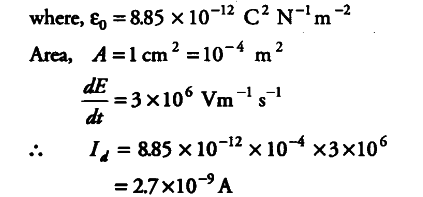# Calculate the displacement

Calculate the displacement current between the square plates of side 1 cm of a capacitor, if electric field between the plates is changing at the rate

We know that,